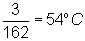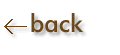Precision, Deviation, Exact Number:

Precision refers to how close several measurements (that have made in the same way) come to each other.

Deviation is the difference between the AVERAGE experimental value obtained and any of the individual readings.

Example:

 Melting Point Deviation 1st Trial 52oC 54 - 52  =  2 2nd Trial 53oC 54 - 53  =  1 3rd Trial 57oC 57 - 54  =  3

Avg. Melting Point:Avg. Deviation:Exact number is a number derived by definition rather than by measurement.    There is no uncertainty in the number.

Example:  12 things  =  1 dozen;    1 meter  =  100 centimeters